# Probability plot correlation coefficient

• Last Updated : 22 Jan, 2021

The probability plot correlation coefficient (PPCC) is a graphical technique for identifying the shape parameter that best describes the dataset. Most of the statistical analysis has been done assuming the shape of the distribution in mind. However, these assumptions may be challenged because sometimes the distributions can have very different shapes depending upon the shape parameter. Therefore, it is better to find the shape parameter as part of the analysis, so that we can be more confident about the distribution of the population.

The PPCC plot is formed using the following axes:

• Vertical Axis: Probability plot correlation coefficient
• Horizontal Axis: Value of shape parameter

The main aim of the PPCC plot is first to find a good value of the shape parameter. In addition to calculating the shape parameter of the distribution, the PPCC plot can be used in deciding which distributional family is most appropriate.

The PPCC plot answers the following questions:

• What is the best-fit member within a distributional family?
• Does this best-fit member generate a good enough fit?
• Does this distributional family provide a good fit compared to other distributions?
• How sensitive is the choice of the shape parameter?

The Turkey-lambda PPCC plot, with shape parameter λ, is particularly useful for symmetric distributions. It indicates whether a distribution is short or long-tailed and it can further indicate several common distributions. Specifically,

• λ =-1, distribution is approximately Cauchy.
• λ = 0, distribution is exactly logistic.
• λ = 0.14, distribution is approximately normal.
• λ = 0.5, distribution is U-shaped.
• λ = 1, distribution is exactly uniform.

If the Turkey-Lambda PPCC plot gives a maximum value = 0.14, then we can conclude that the normal distribution is good approximate for the data. If the maximum value is < 0.14 then it means a long-tailed distribution such as the double exponential or logistic would be a better choice. If the maximum value is -1, then it implies a very-long tailed distribution such as Cauchy. If the maximum value is > 0.14 then it implies a very short-tailed distribution such as Beta or Uniform.

### Implementation

• In this implementation, we will be generating different distribution and checking their Turkey-Lambda shape parameter value, and plotting PPCC plots. I am using Google Colaboratory, which contains some pre-installed libraries such as scipy, numpy, statsmodel, seaborn etc. However, these libraries can be easily installed using pip install in the local environment.

## Python3

 `# import libraries ` `import` `numpy as np ` `import` `matplotlib.pyplot as plt ` `import` `scipy.stats as sc ` `import` `seaborn as sns ` ` `  `# generate different distributions ` `sample_size ``=` `10000`  `standard_norm ``=` `np.random.normal(size``=``sample_size) ` `cauchy_dist ``=` `sc.cauchy.rvs(loc``=``1``, scale``=``10``, size``=``sample_size) ` `logistic_dist ``=` `np.random.logistic(size``=``sample_size) ` `uniform_dist ``=` `np.random.uniform(size``=` `sample_size) ` `beta_dist ``=` `np.random.beta(a``=``1``, b``=``1``, size``=``sample_size) ` ` `  `# Normal Distribution ` `fig, ax ``=` `plt.subplots(``1``, ``2``, figsize``=``(``12``, ``7``)) ` `sns.histplot(standard_norm,kde``=``True``, color ``=``'blue'``,ax``=``ax[``0``]) ` `sc.ppcc_plot(standard_norm, ``-``5``,``5``, plot``=``ax[``1``]) ` `shape_param_normal ``=` `sc.ppcc_max(standard_norm) ` `ax[``1``].vlines(shape_param_normal,``0``,``1``, colors``=``'red'``) ` `print``(``"shape parameter of normal distribution is "``, shape_param_normal) ` ` `  `# Cauchy Distribution ` `fig, ax ``=` `plt.subplots(``1``, ``2``, figsize``=``(``12``, ``7``)) ` `sns.histplot(cauchy_dist, color ``=``'blue'``,ax``=``ax[``0``]) ` `ax[``0``].set_xlim(``-``40``,``40``) ` `sc.ppcc_plot(cauchy_dist, ``-``5``,``5``, plot``=``ax[``1``]) ` `shape_param_cauchy ``=` `sc.ppcc_max(cauchy_dist) ` `ax[``1``].vlines(shape_param_cauchy,``0``,``1``, colors``=``'red'``) ` `print``(``'shape parameter of cauchy distribution is '``,shape_param_cauchy) ` ` `  `# Logistic Distribution ` `fig, ax ``=` `plt.subplots(``1``, ``2``, figsize``=``(``12``, ``7``)) ` `sns.histplot(logistic_dist, color ``=``'blue'``,ax``=``ax[``0``]) ` `sc.ppcc_plot(logistic_dist, ``-``5``,``5``, plot``=``ax[``1``]) ` `shape_param_logistic ``=` `sc.ppcc_max(logistic_dist) ` `ax[``1``].vlines(shape_param_logistic,``0``,``1``, colors``=``'red'``) ` `print``(``"shape parameter of logistic is "``,shape_param_logistic) ` ` `  `# Uniform Distribution ` `fig, ax ``=` `plt.subplots(``1``, ``2``, figsize``=``(``12``, ``7``)) ` `sns.histplot(uniform_dist, color ``=``'green'``,ax``=``ax[``0``]) ` `sc.ppcc_plot(uniform_dist, ``-``5``,``5``, plot``=``ax[``1``]) ` `shape_para_uniform ``=``sc.ppcc_max(uniform_dist) ` `ax[``1``].vlines(shape_para_uniform,``0``,``1``, colors``=``'red'``) ` `print``(``"shape parameter of uniform distribution is "``,shape_para_uniform) ` ` `  `# Beta Distribution ` `fig, ax ``=` `plt.subplots(``1``, ``2``, figsize``=``(``12``, ``7``)) ` `sns.histplot(beta_dist, color ``=``'blue'``,ax``=``ax[``0``]) ` `sc.ppcc_plot(beta_dist, ``-``5``,``5``, plot``=``ax[``1``]) ` `shape_para_beta ``=``sc.ppcc_max(beta_dist) ` `ax[``1``].vlines(shape_para_beta,``0``,``1``, colors``=``'red'``) ` `print``(``"shape parameter of beta distribution is :"``,shape_para_beta)`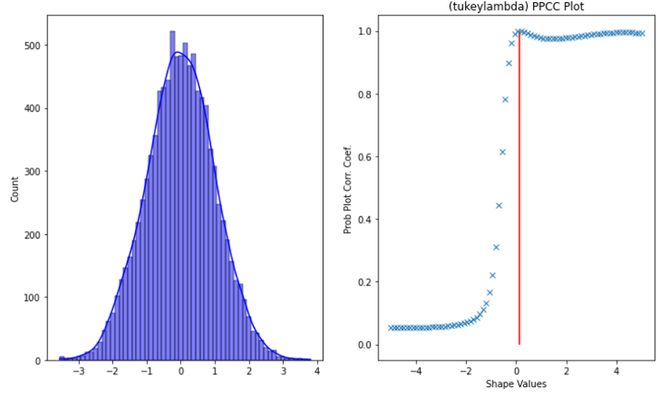Normal Distribution with PPCC plot

`shape parameter of normal distribution is  0.14139046072745928`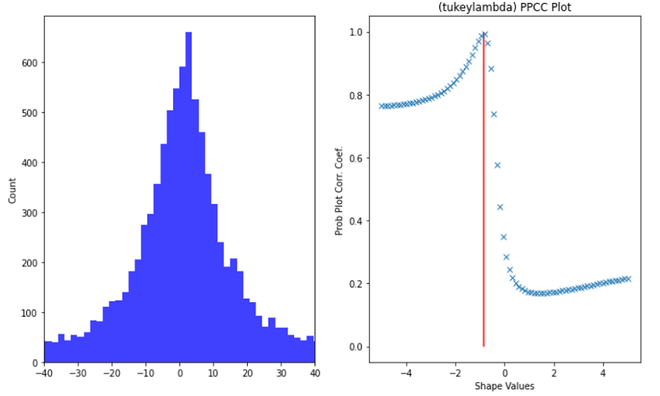Cauchy Distribution with PPCC plot

`shape parameter of cauchy distribution is  -0.8555566289941865`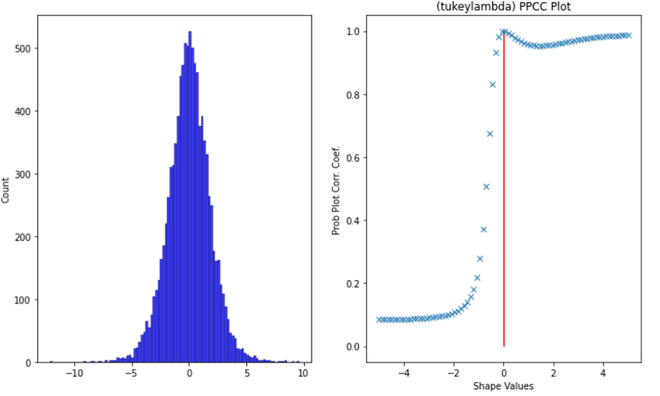Logistic Distribution with PPCC plot

`shape parameter of logistic is  0.003792036190661425`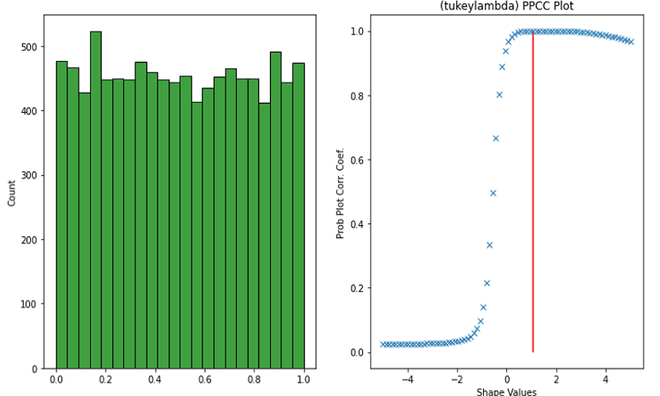Uniform distribution with PPCC plot

`shape parameter of uniform distribution is  1.0681942803525217`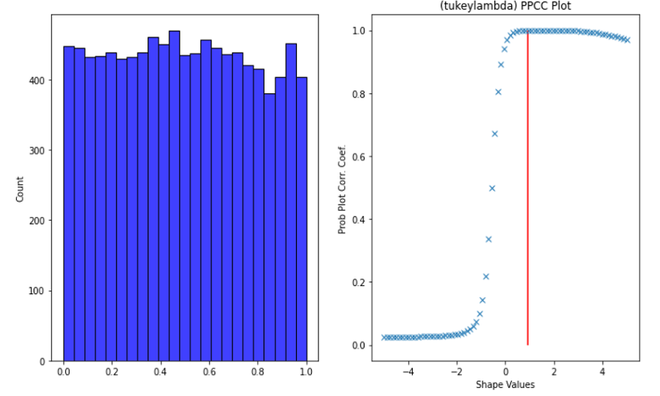Beta distribution with PPCC plot

`shape parameter of beta distribution is : 0.9158983492057267`

#### References

My Personal Notes arrow_drop_up
Recommended Articles
Page :